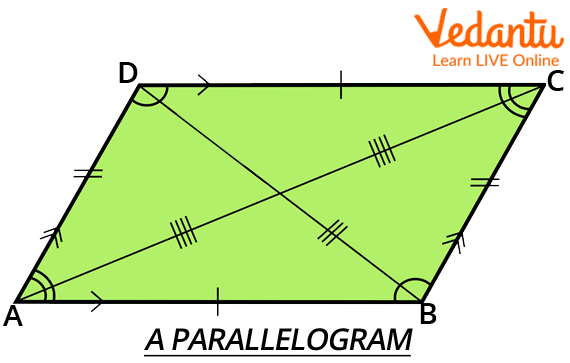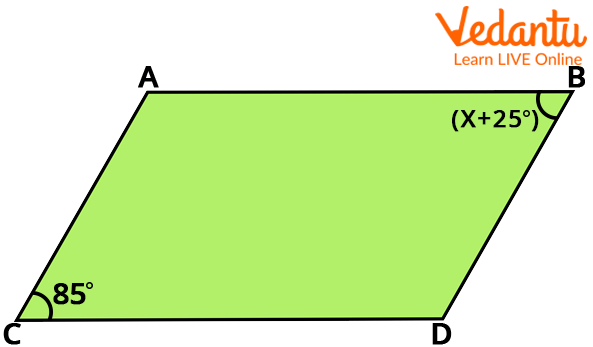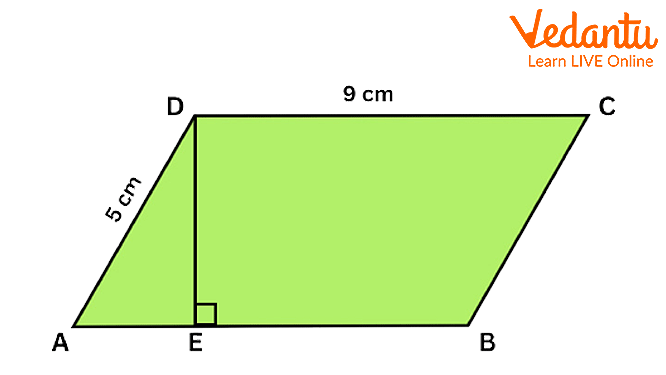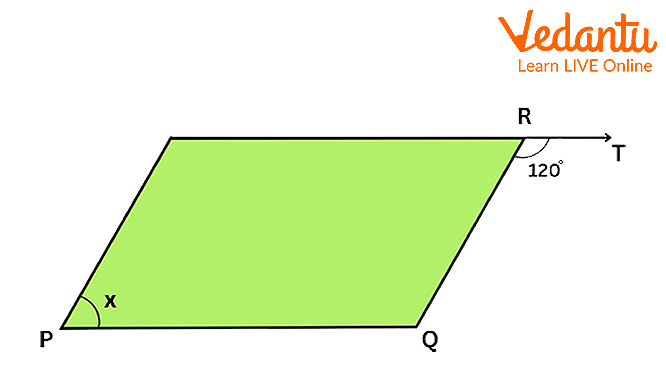Courses
Courses for Kids
Free study material
Free LIVE classes
More

# Opposite Sides are EqualLIVE
Join Vedantu’s FREE Mastercalss

## Introduction

The magnitude (modulum) of the product of two adjacent vectors, which are thought of as vectors, determines the area of a parallelogram. A parallelogram's opposing sides are of equal length (congruent). In a parallelogram, the opposing angles are equal in size. Two adjacent vertices' angles can be any multiple of 180 degrees. Each parallelogram's inside angles add up to 360° in total.

In today's lesson, we will show that the opposite sides of a parallelogram are equal.

A parallelogram is a quadrilateral where the two opposite sides are parallel. We will show that, in that case, they are also equal.

### What is Parallelogram

A parallelogram is a geometric object with sides that are parallel to one another in two dimensions. It is a form of polygon with four sides (sometimes known as a quadrilateral) in which each parallel pair of sides have the same length. A parallelogram has neighbouring angles that add up to 180 degrees. You must have studied a variety of 2D forms and sizes in geometry, including circles, squares, rectangles, rhombuses, etc. Each of these forms has a unique set of characteristics.A Parallelogram

### Definition

A quadrilateral having two sets of parallel sides is referred to as a parallelogram. In a parallelogram, the opposing sides are of equal length, and the opposing angles are of equal size. Additionally, the interior angles that are additional to the transversal on the same side. 360 degrees is the sum of all interior angles.

A parallelepiped is a three-dimensional shape with parallelogram-shaped faces. The base (one of the parallel sides) and height (the distance from top to bottom) of the parallelogram determine its area. A parallelogram's perimeter is determined by the lengths of its four sides.

The qualities of a parallelogram are shared by the forms of a square and a rectangle.

### Theorem of Parallelogram

The forms that result from moving from one point to the next while holding a pencil with a sharp tip on a piece of paper are known as plane curves. A simple curve is one that does not cross itself at any point. A polygon is a straightforward closed planar curve consisting entirely of line segments.

A parallelogram is a polygon. A parallel quadrilateral with both sets of opposing sides is known as a parallelogram. We shall gain in-depth information on the theorems and characteristics of parallelograms in this post.

Theorem : In a parallelogram, opposite sides are equal.

Given:

Solution: Let ABCD be a parallelogram and AC be a diagonal.

Observe that the diagonal AC divides parallelogram ABCD into two triangles: $\Delta ABC$ and$\Delta CDA$.

We need to first prove that these triangles are congruent.

In $\Delta ABC$ and $\Delta CDA$; note that $BC{\rm{ }}||{\rm{ }}AD$ and AC is a transversal.

So, $BCA{\rm{ }} = {\rm{ }}DAC$ (Pair of alternate angles)

And $AC{\rm{ }} = {\rm{ }}CA$ (common)

So, $\Delta ABC$ and $\Delta CDA$; are congruent (ASA rule).

Therefore, the corresponding parts $AB{\rm{ }} = {\rm{ }}CD$ and $AD{\rm{ }} = {\rm{ }}BC$

Hence proved.Parallelogram Theorem

### Solved Questions

1. Find the area of parallelogram ABCD if AE : EB = 1 : 2.Question 1

a) 25 sqm

b) 22.5 sqm

c) 36 sqm

d) 45 sqm

Explanation: In parallelogram ABCD, AB = CD = 9cm  (Opposite sides of a parallelogram are equal)

Now, $AE{\rm{ }}:{\rm{ }}EB{\rm{ }} = {\rm{ }}1{\rm{ }}:{\rm{ }}2$and $AE{\rm{ }} + {\rm{ }}EB{\rm{ }} = {\rm{ }}AB$

$\Rightarrow k{\rm{ }} + {\rm{ }}2k{\rm{ }} = {\rm{ }}9 \Rightarrow k{\rm{ }} = {\rm{ }}3$

$\Rightarrow AE{\rm{ }} = {\rm{ }}3cm$

In $\Delta AED,{\rm{ }}A{D^2}\; = {\rm{ }}A{E^2}\; + {\rm{ }}E{D^2}$  (By Pythagoras theorem)

$\Rightarrow {5^2}\; = {\rm{ }}{3^2}\; + {\rm{ }}E{D^2}$

$\Rightarrow E{D^2}\; = {\rm{ }}25{\rm{ }}--{\rm{ }}9{\rm{ }} = {\rm{ }}16$

$\Rightarrow ED{\rm{ }} = {\rm{ }}4cm$

Now, Area of parallelogram ABCD = Base x corresponding altitude $= {\rm{ }}AB{\rm{ }} \times {\rm{ }}DE{\rm{ }} = {\rm{ }}9{\rm{ }} \times 4{\rm{ }} = {\rm{ }}36sqm.$

2. In the parallelogram ABCD, $\angle ABC{\rm{ }} = {\rm{ }}50^\circ$. Find the measures of $\angle BCD,\angle CBA$ and $\angle DAB.$

Solution:

As$AB\parallel DC,\angle ABC{\rm{ }} + \angle BCD{\rm{ }} = {\rm{ }}180^\circ$

Therefore, $\angle BCD{\rm{ }} = {\rm{ }}180^\circ - \;\angle ABC$

$= {\rm{ }}180^\circ {\rm{ }} - \;50^\circ$

$= \;130^\circ$

As opposite angles in a parallelogram are equal,

$\angle CDA{\rm{ }} = \angle ABC{\rm{ }} = {\rm{ }}50^\circ$ and

$\angle DAB{\rm{ }} = \angle BCD{\rm{ }} = {\rm{ }}130^\circ$

3. Find the value of x if PQRS is a parallelogram.Question 3

a) $150^\circ$

b) $50^\circ$

c) $60^\circ$

d) $120^\circ$

Explanation: $\angle SRQ{\rm{ }} + \angle QRT{\rm{ }} = {\rm{ }}180^\circ$ (Linear Pair)
$\Rightarrow \angle SRQ{\rm{ }} = {\rm{ }}60^\circ$
In parallelogram PQRS, $\angle SRQ{\rm{ }} = \angle QPS$  (Opposite angles of a parallelogram are equal)
$\Rightarrow x{\rm{ }} = {\rm{ }}60^\circ .$

### Conclusion

This article covered our study of the parallelogram. A parallelogram is a quadrilateral with two pairs of parallel sides. A parallelogram has opposite sides of the same length and angles of the same size. the internal angles on the same side that are in addition to the transversal. The total of all internal angles is 360 degrees. And the theorem that establishes the equality of the opposing sides of a parallelogram.

Last updated date: 28th Sep 2023
Total views: 42.9k
Views today: 1.42k

## FAQs on Opposite Sides are Equal

1. Give a few real-life examples of a Parallelogram.

The real-life examples of a Parallelogram are

1. Tiles

2. Buildings

3. Roofs

4. Paper

5. Desks

6. Erasers

7. Solar Panels

8. Striped Pole

9. Steps of a Staircase

10. Design on a Cardigan

2. Mention any 2 Properties of Parallelogram?

Properties of Parallelogram

1. The opposite sides of a parallelogram are parallel and equal in length.

2. The opposite angles of a parallelogram are congruent.

3. The sum of the adjacent angles of a parallelogram is equal to 180°. Hence, the consecutive angles of a parallelogram are supplementary.

3. Do parallelograms opposite angles are equal?

Yes, opposite sides of a parallelogram are equal.